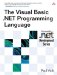# VBMath Module

 <  Day Day Up  >

The VBMath module contains useful mathematical functions and subroutines.

## Compatibility

Most of the members of the Math module in previous versions of Visual Basic have moved to the System.Math class.

` Public Sub Randomize() Public Sub Randomize(ByVal Number As Double) `

The Randomize subroutine seeds the Rnd function's random number generator. If a seed is not specified, the current time is used as the seed.

` Public Function Rnd() As Single Public Function Rnd(ByVal Number As Single) As Single `

The Rnd function returns a random floating-point number greater than or equal to 0, but less than 1. If the Number argument is supplied, the behavior of the function depends on the value of Number . If Number is less than 0, Rnd returns a random number using Number as the seed. If Number is 0, Rnd returns the most recent random number returned by Rnd . If Number is greater than 0, Rnd returns a new random number. To produce a random number between a lower bound (e.g., 0) and an upper bound (e.g., 10), you can use the expression CInt(Int((upperbound - lowerbound + 1) * Rnd() + lowerbound)) .

## NOTE

The .NET Framework class System.Random can also be used to generate random numbers . The Rnd function and the Random class use different random number generation algorithms, so each one will generate a different sequence of random numbers, given the same starting seed.

 <  Day Day Up  >The Visual Basic .NET Programming Language
ISBN: 0321169514
EAN: 2147483647
Year: 2004
Pages: 173
Authors: Paul Vick

Similar book on Amazon